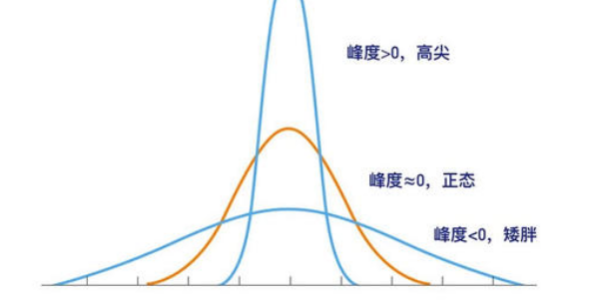• 至于具体的函数形式，由所考察总体固有的特征来决定的。总体归函数(Population regression function)计量经济学用语，表明被解释变量Y的平均状态(总体条件期望)随解释变量X变化的规律。扩展资料：二项...
系数由数据计算出来的到，可以推出其是自变量和误差的线性函数，因为误差服从正态分布，故也服从正态分布。总体归函数表明被解释变量Y的平均状态(总体条件期望)随解释变量X变化的规律。至于具体的函数形式，是由所考察总体固有的特征来决定的。总体归函数(Population regression function)计量经济学用语，表明被解释变量Y的平均状态(总体条件期望)随解释变量X变化的规律。扩展资料：二项分布介绍：二项分布是由伯努利提出的概念，指的是重复n次独立的伯努利试验。在每次试验中只有两种可能的结果，而且两种结果发生与否互相对立，并且相互独立，与其它各次试验结果无关，事件发生与否的概率在每一次独立试验中都保持不变，则这一系列试验总称为n重伯努利实验，当试验次数为1时，二项分布服从0-1分布。在概率论和统计学中，二项分布是n个独立的是/非试验中成功的次数的离散概率分布，其中每次试验的成功概率为p。这样的单次成功/失败试验又称为伯努利试验。参考资料来源：百度百科-二项分布
展开全文• 目录什么是LDA主题模型背景知识贝叶斯理论gamma函数多个分布博鲁尼分布二项分布多项分布beta分布Dirichlet 分布开始了解LDAPython建模 什么是LDA主题模型 首先说明一下什么是主题模型。这里主题模型把一份份不同...
目录什么是LDA主题模型背景知识贝叶斯理论gamma函数多个分布博鲁尼分布二项分布多项分布beta分布Dirichlet 分布开始了解LDAPython建模
什么是LDA主题模型
首先说明一下什么是主题模型。这里的主题模型是把一份份不同的文本内容通过某种方式来找到这些文本对应的主题。打个比方：我有一堆新闻类文档，但我想将这对文档进行主题分类。到底是娱乐？军事？政治？等主题。这时候就使用到主题模型。
而这里的LDA和特征工程的LDA不一样。全名Latent Dirichlet Allocation，是一款基于Dirichlet分布的概率主题模型。他属于非监督学习，当中运用到了概率论以及词袋模型等知识。
背景知识
为了更容易的了解LDA的工作原理，请原谅我的啰嗦。先简单的科普一下背景知识。当然已经熟悉的朋友，可以选择忽略这个酌情下一步
贝叶斯理论
这个应该很多人都认识的。这里面先验概率等知识我就不说了。这里先列出贝叶斯公式：
$P(\theta,y) = P(\theta)P(y|\theta)$
然后就演变出
$P(\theta|y) = \frac{P(\theta,y)}{ P(\theta)}=\frac{P(\theta)P(y|\theta)}{P(\theta)}$
这个公式的伟大之处就是实现了概率统计上的“交换律”。本来我们要求y下发现的概率，变成了theta下的概率。这为后面LDA模型提供了基础
gamma函数
gamma是阶乘函数在实数的推广。先回顾一下实数的阶乘公式
$\Gamma(n) = (n-1)!$
相对于实数界的有
$\Gamma(x) = \int_0^\infty{t^{x-1}}e^{-t}dt$
多个分布
博鲁尼分布
博鲁尼就是只有两种出现的情况。比如要么0，要么1。我制作一次实现得到的分布结果。
二项分布
二项分布就是某一种概率事件，他只可能出现两种情况，要么正要么负{+，-}。他是博鲁尼分布执行N此以后的结果。这种分布就是二项分布。比如最常见的就是抛硬币，出现字和出现图案的概率就是二项分布。二项分布的密度函数有
$P(K=k)=(^n_k)p^k(1-p)^{n-k}$
多项分布
多项分布就是二项分布的多维度展开式。也就是说我的概率事件不在是{+，-}。而是存在很多种情况。比如掷骰子，概率事件有{1,2,3,4,5,6}。在执行N此实验以后，就会出现k=6的多项分布。相关密度函数有
$P(x_1,x_2,···,x_k;n,p_1,p_2,···,p_k)=\frac{n!}{x_1!···x_n!}P_1^{X_1}···P_n^{X_n}$
beta分布
beta分布式二项分布的共轭先验分布。说白了他就是二项分布+gamma函数。
给定参数a他的函数公式有：
$Beta(x|\alpha,\beta)=\frac{\Gamma(a+\beta)}{\Gamma(a)\Gamma(\beta)}p^{\alpha-1}(1-x)^{\beta-1}$
Dirichlet 分布
最后Dirichlet分布其实就是beta分布的多维度推广。他们的关系可以理解为二项分布与多项分布的关系。所以，他的函数公式为：
$Dirichlet(x_1,x_2,···,x_n;\alpha_1,\alpha_2,···,\alpha_n)=\frac{\Gamma(\alpha_1+\alpha_2+···+\alpha_n)}{\Gamma(\alpha_1)\Gamma(\alpha_2)···\Gamma(\alpha_n)}x_1^{\alpha_1-1}x_2^{\alpha_2-1}···x_n^{\alpha_n-1}$
这里细看一下，是不是感觉和多项式分布的公式有点像
开始了解LDA
上面讲了那么多东西，那么到底什么才是LDA模型呢？我们先拟定一个命题：现在我们有很多篇新闻稿件，我们要针对这些稿件进行主题分类。大概如图下面的意思（图片来源于网络）也就是我们需要求概率P(主题|文档)。然后从P的最大值获取该稿件的最可能概率。这里利用贝叶斯概率公式的链条化简，可以得到这么一条公式
$P(单词|文档)=P(单词|主题)P(主题|文档)$
具体推导我这里就不列出来了，大家可以自行对照上面的贝叶斯进行化简。
从这里我们可以看出，LDA对主题分类做了一件什么事呢？他其实就是求P(单词|文档) 和 P(单词|主题) 这两个概率分布，然后结合着两个概率分布来求出P(主题|文档)的最大值。最后得到该文档的主题。所以LDA的具体流程如下：他的具体步骤如下：

首先假设稿件主题的先验分布服从Dirichlet分布。既对某个文档d，其主题的分布有：
$\theta_{d}=Dirichlet(\alpha)$
这里对应的是流程图中左边的两个圈圈的步骤。

假设主题中的词的先验分布服从Dirichlet分布。既对任意一个主题k有：
$\beta_{k}=Dirichlet(\mu)$
这里对应流程图中右边的两个圈圈。

最后结合两边的分布最后得到最后的词分布，从而达到预测主题的想过。

Python建模
理论知识就到此为止，接下来我们进行python的建模。采用往上面比较多用的希拉里邮件数据：https://wx.jdcloud.com/market/packet/10487
然后下面是我的代码和注释
# 导包
import numpy as np
import pandas as pd
import re
from gensim import corpora, models, similarities
import gensim

# 文本预处理
def clean_email_text(text):
text = text.replace('\n'," ") #新行，我们是不需要的
text = re.sub(r"-", " ", text) #把 "-" 的两个单词，分开。（比如：july-edu ==> july edu）
text = re.sub(r"\d+/\d+/\d+", "", text) #日期，对主体模型没什么意义
text = re.sub(r"[0-2]?[0-9]:[0-6][0-9]", "", text) #时间，没意义
text = re.sub(r"[\w]+@[\.\w]+", "", text) #邮件地址，没意义
text = re.sub(r"/[a-zA-Z]*[:\//\]*[A-Za-z0-9\-_]+\.+[A-Za-z0-9\.\/%&=\?\-_]+/i", "", text) #网址，没意义
pure_text = ''
# 以防还有其他特殊字符（数字）等等，我们直接把他们loop一遍，过滤掉
for letter in text:
# 只留下字母和空格
if letter.isalpha() or letter==' ':
pure_text += letter
# 再把那些去除特殊字符后落单的单词，直接排除。
# 我们就只剩下有意义的单词了。
text = ' '.join(word for word in pure_text.split() if len(word)>1)
return text
# 数据读取
# 简单处理一下，去掉空
df = df[['Id','ExtractedBodyText']].dropna()
# 清除无用内容，只保留单词
docs = df['ExtractedBodyText'].apply(lambda x: clean_email_text(x))
doclist = docs.values
# 手动列出停用词列表，中文的话可稍微百度一下虚词列表
stoplist = ['very', 'ourselves', 'am', 'doesn', 'through', 'me', 'against', 'up', 'just', 'her', 'ours',
'couldn', 'because', 'is', 'isn', 'it', 'only', 'in', 'such', 'too', 'mustn', 'under', 'their',
'if', 'to', 'my', 'himself', 'after', 'why', 'while', 'can', 'each', 'itself', 'his', 'all', 'once',
'herself', 'more', 'our', 'they', 'hasn', 'on', 'ma', 'them', 'its', 'where', 'did', 'll', 'you',
'didn', 'nor', 'as', 'now', 'before', 'those', 'yours', 'from', 'who', 'was', 'm', 'been', 'will',
'into', 'same', 'how', 'some', 'of', 'out', 'with', 's', 'being', 't', 'mightn', 'she', 'again', 'be',
'by', 'shan', 'have', 'yourselves', 'needn', 'and', 'are', 'o', 'these', 'further', 'most', 'yourself',
'having', 'aren', 'here', 'he', 'were', 'but', 'this', 'myself', 'own', 'we', 'so', 'i', 'does', 'both',
'when', 'between', 'd', 'had', 'the', 'y', 'has', 'down', 'off', 'than', 'haven', 'whom', 'wouldn',
'should', 've', 'over', 'themselves', 'few', 'then', 'hadn', 'what', 'until', 'won', 'no', 'about',
'any', 'that', 'for', 'shouldn', 'don', 'do', 'there', 'doing', 'an', 'or', 'ain', 'hers', 'wasn',
'weren', 'above', 'a', 'at', 'your', 'theirs', 'below', 'other', 'not', 're', 'him', 'during', 'which','am','pm']
# 去除停用词
texts = [[word.lower() for word in doc.lower().split() if word not in stoplist] for doc in doclist]
# 创建语料库，把每一个单词转化成元祖模式展示[(15, 1), (17, 1)]
dictionary = corpora.Dictionary(texts)
corpus = [dictionary.doc2bow(text) for text in texts]
# 训练模型
lda = gensim.models.ldamodel.LdaModel(corpus=corpus,id2word=dictionary,num_topics=20)
# 打印主题各单词结果
print(lda.print_topics(num_topics=20,num_words=10))
# 保存语料库与模型
lda.save('hillary_classify_model.model')
dictionary.save('hillary_classify_dictionary.model')
# 模型的加载和预测
# 这里假设我有一篇关于奥巴马与民主党的新闻稿件
s = 'The news of Obama or Republican Party'
text = s.lower().split(' ')
# 预测主题
bow = dic.doc2bow(text)
model.get_document_topics(bow)




展开全文python 机器学习 自然语言处理 nlp LDA主题模型
• 六、为什么Logistic回归输入特征一般都离散化而不是连续？ 七、Logistic回归和SVM关系 一、Logistic分布 定义：X连续随机变量，X服从logistic分布，则X具有下列的分布函数和密度函数： 其中，μ为位置...
目录：
一、Logistic分布
二、二项Logistic回归原理
三、参数估计
四、Logistic回归的正则化
五、Logistic回归和线性回归区别
六、为什么Logistic回归的输入特征一般都是离散化而不是连续的？
七、Logistic回归和SVM的关系
一、Logistic分布
定义：X是连续随机变量，X服从logistic分布，则X具有下列的分布函数和密度函数：其中，μ为位置参数，γ为形状参数曲线在中心附近增长速度较快，并且γ值越小，曲线在中心附近的增长速度越快。
特别的，当μ=0，γ=1的时候就是sigmoid函数。
二、二项Logistic回归原理
二项Logistic回归模型时一种分类模型，由条件概率分布P(Y|X)表示，随机变量Y取0或1。
定义二项logistic回归模型的条件分布如下：其中x∈Rn是输入，Y∈{0,1}是输出，W∈Rn和b∈R是参数，w称为权重，b称为偏置。
有时为了方便会将权重向量和输入向量进行扩充：
w = (w1,w2, …, wn, b)T，x = (x1,x2, …, xn, 1)T
所以，logistic回归模型变为：得到概率之后，我们可以通过设定一个阈值将样本分成两类。如：阈值为0.5的时候，当大于0.5则为一类，小于0.5为另一类。
三、参数估计
有了以上的模型，我们就需要对模型中的参数w求出来。我们可以使用极大似然估计法估计模型的参数。
设：似然函数为：对数似然函数：对L(w)求极大值，得到w的估计值。通常采用梯度下降法或拟牛顿法求解参数w。
四、Logistic回归的正则化
正则化是为了解决过拟合问题。分为L1和L2正则化。目标函数中加入正则化，即加入模型复杂性的评估。正则化符合奥卡姆剃刀原理，即：在所有可能的模型中，能够很好的解释已知数据并且十分简单的模型才是最好的模型。
加入正则化后，模型的目标函数变为：P表示范数，p=1为L1正则化，p=2为L2正则化
L1正则化：向量中各元素绝对值的和。关键在于能够对特征进行自动选择，稀疏参数可以减少非必要的特征引入噪声。
L2正则化：向量中个元素的平方和，L2会使得各元素尽可能小，但都不为零。左边为L1正则化，右边为L2正则化。假设权重参数w只有二维w1和w2。L1为各元素绝对值和，即|w1|+|w2| = C，则得到的形状为棱形，L2为(w1)2+(w2)2 = C，则形状为圆。很容易可以发现L1更容易在顶点处相切，L2则不容易在顶点处相切。顶点处则其中一个参数为0，这就是为什么L1会使得参数稀疏的原因。
五、Logistic回归和线性回归区别

Logistic回归在线性回归的实数输出范围加上sigmoid函数，将输出值收敛在0~1之间。其目标函数也因此从差平方和函数变为对数损失函数。

逻辑回归和线性回归都是广义的线性回归，线性回归是使用最小二乘法优化目标函数，而逻辑回归是使用梯度下降或者拟牛顿法。

线性回归在整个实数域范围内进行预测，敏感度一致，而分类范围需要在[0,1]。逻辑回归是一种减少预测范围，将预测值限定为[0,1]间的一种回归模型。因而对于二分类问题，逻辑回归的鲁棒性更好。

逻辑回归是以线性回归为理论支持的，但线性回归模型无法做到sigmoid的非线性形式。Sigmoid可以轻松处理0/1分类问题。

六、为什么Logistic回归的输入特征一般都是离散化而不是连续的？

离散特征容易增加和减少，使得模型容易迭代。

离散特征的内积运算速度快，计算结果方便存储。

对异常值不敏感，比如一个特征是年龄>30为1，否则为0，如果特征没有离散化。一个异常数据300岁会给模型带来很大的干扰。

逻辑回归是广义线性模型，表达能力受限。单变量离散化为N个后，每个变量都有单独的权重，相当于为模型引入了非线性，能够提升模型的表达能力，加大拟合。

特征离散化后可以进行特征交叉，由M+N变量变为M*N个变量，进一步引入非线性，提升表达能力。

特征离散化后，模型会更加稳定。比如对用户年龄离散化，将20~30作为一个区间，这样不会因为一个用户年龄大了一岁就变成完全不同的人了，当然处于区间相邻处的样本就刚好相反，所以怎么划分区间是们学问。

特征离散化后，起到了简化了逻辑回归模型的作用，降低了模型过拟合的风险。

七、Logistic回归和SVM的关系

LR和SVM都可以处理分类问题，且一般都处理线性二分类问题。

LR是参数模型，SVM是非参数模型。

LR的目标函数是对数似然函数，SVM的目标函数是hinge损失函数。这两个函数都是增加对分类结果影响较大的数据点的权重，减少影响较小的数据点的权重。

SVM的处理方法是只考虑support vectors，也就是和分类最相关的少数点，去学习分类器。而逻辑回归通过非线性映射，大大减小了离分类平面较远的点的权重，相对提升了与分类最相关的数据点的权重。

逻辑回归相对来说模型更简单，好理解，特别是大规模线性分类时比较方便。而SVM的理解和优化相对来说复杂一些，SVM转化为对偶问题后,分类只需要计算与少数几个支持向量的距离,这个在进行复杂核函数计算时优势很明显,能够大大简化模型和计算。

logic能做的svm能做，但可能在准确率上有问题，svm能做的logic有的做不了。

代码部分
基础函数库
import numpy as np
导入画图库
import matplotlib.pyplot as plt
import seaborn as sns
导入逻辑回归模型函数
from sklearn.linear_model import LogisticRegression
##Demo演示LogisticRegression分类
构造数据集
x_fearures = np.array([[-1, -2], [-2, -1], [-3, -2], [1, 3], [2, 1], [3, 2]])
y_label = np.array([0, 0, 0, 1, 1, 1])
调用逻辑回归模型
lr_clf = LogisticRegression()
用逻辑回归模型拟合构造的数据集
lr_clf = lr_clf.fit(x_fearures, y_label) #其拟合方程为 y=w0+w1x1+w2x2
##查看其对应模型的w
print(‘the weight of Logistic Regression:’,lr_clf.coef_)
##查看其对应模型的w0
print(‘the intercept(w0) of Logistic Regression:’,lr_clf.intercept_)
##the weight of Logistic Regression:[[0.73462087 0.6947908]]
##the intercept(w0) of Logistic Regression:[-0.03643213]
可视化构造的数据样本点
plt.figure()
plt.scatter(x_fearures[:,0],x_fearures[:,1], c=y_label)#画出散点图
plt.title(‘Dataset’)#设置标题
plt.show()#展示结果
nx, ny = 200, 100#用来生成网格
x_min, x_max = plt.xlim()#图中X轴展示的最小和最大值
y_min, y_max = plt.ylim()
x_grid, y_grid = np.meshgrid(np.linspace(x_min, x_max, nx),np.linspace(y_min, y_max, ny))#x_grid 生成100个   将横坐标平分成200分，的一个数值列表  y_grid 生成200个100
plt.show()
z_proba = lr_clf.predict_proba(np.c_[x_grid.ravel(), y_grid.ravel()])#取出一个每一个点，预测属于每一个种类的概率
z_proba = z_proba[:, 0].reshape(y_grid.shape)
plt.contour(x_grid, y_grid, z_proba, [0.5], linewidths=2., colors=‘blue’)#将决策边界的点显示出来即概率为0.5的点
plt.show()
可视化预测新样本
#生成画布
plt.figure()
new point 1
x_fearures_new1 = np.array([[0, -1]])
plt.scatter(x_fearures_new1[:,0],x_fearures_new1[:,1], s=50, cmap=‘viridis’)
plt.annotate(s=‘New point 1’,xy=(0,-1),xytext=(-2,0),color=‘blue’,arrowprops=dict(arrowstyle=’-|>’,connectionstyle=‘arc3’,color=‘red’))
new point 2
x_fearures_new2 = np.array([[1, 2]])
plt.scatter(x_fearures_new2[:,0],x_fearures_new2[:,1], s=50, cmap=‘viridis’)
plt.annotate(s=‘New point 2’,xy=(1,2),xytext=(-1.5,2.5),color=‘red’,arrowprops=dict(arrowstyle=’-|>’,connectionstyle=‘arc3’,color=‘red’))
训练样本
plt.scatter(x_fearures[:,0],x_fearures[:,1], c=y_label, s=50, cmap=‘viridis’)
plt.title(‘Dataset’)
可视化决策边界
plt.contour(x_grid, y_grid, z_proba, [0.5], linewidths=2., colors=‘blue’)
plt.show()


展开全文